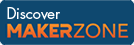Cody

# Problem 51. Find the two most distant points

Created by Cody Team in Community

Given a collection of points, return the indices of the rows that contain the two points most distant from one another. The input vector p has two columns corresponding to the x and y coordinates of each point. Return ix, the (sorted) pair of indices pointing to the remotest rows. There will always be one unique such pair of points.

So if

``` p = [0 0]
[1 0]
[2 2]
[0 1]```

Then

` ix = [1 3]`

That is, the two points p(1,:) and p(3,:) are farthest apart.

### Solution Stats

50.26% Correct | 49.74% Incorrect
Last solution submitted on Mar 18, 2019

#### TagsMATLAB and Simulink resources for Arduino, LEGO, and Raspberry Pi